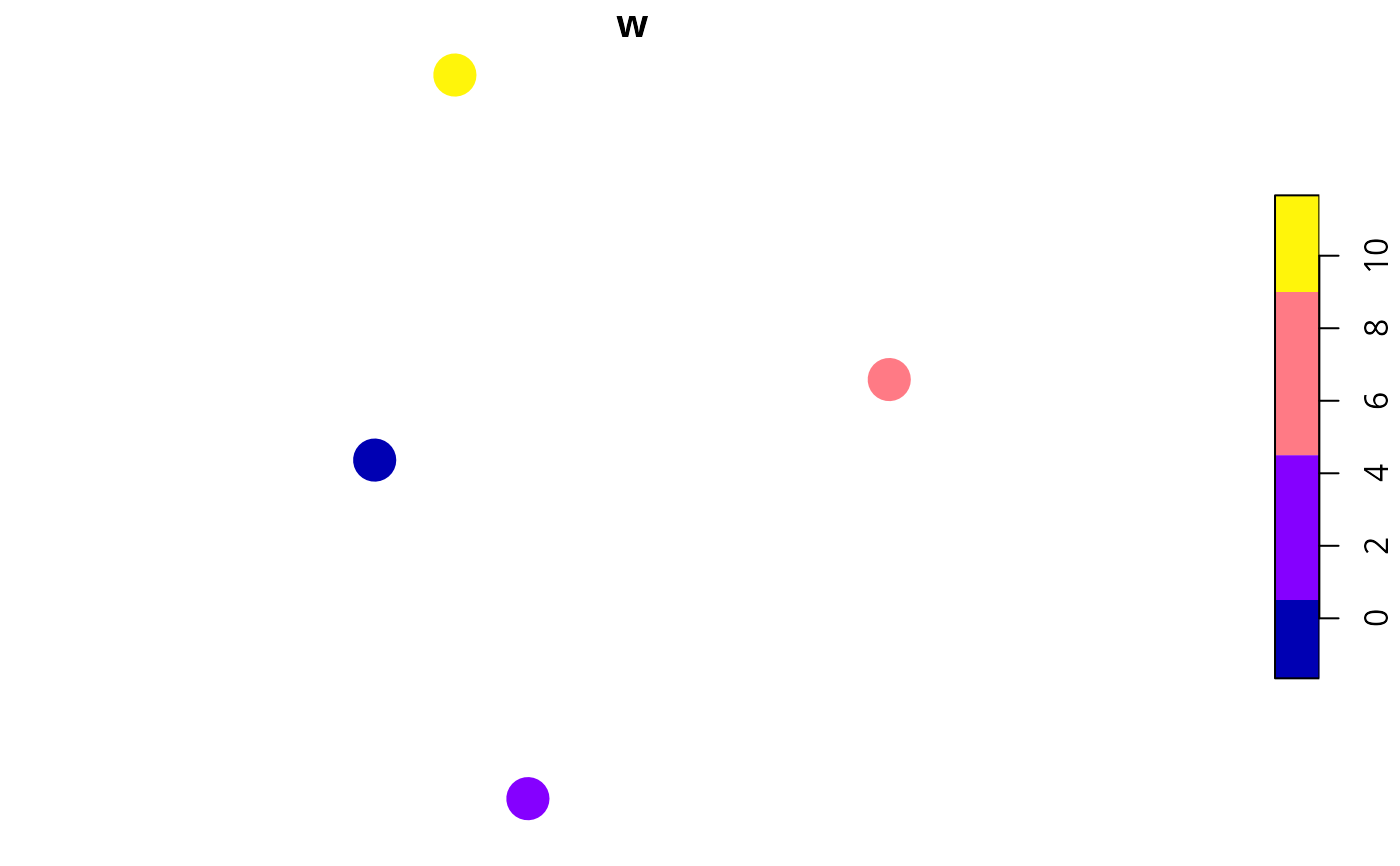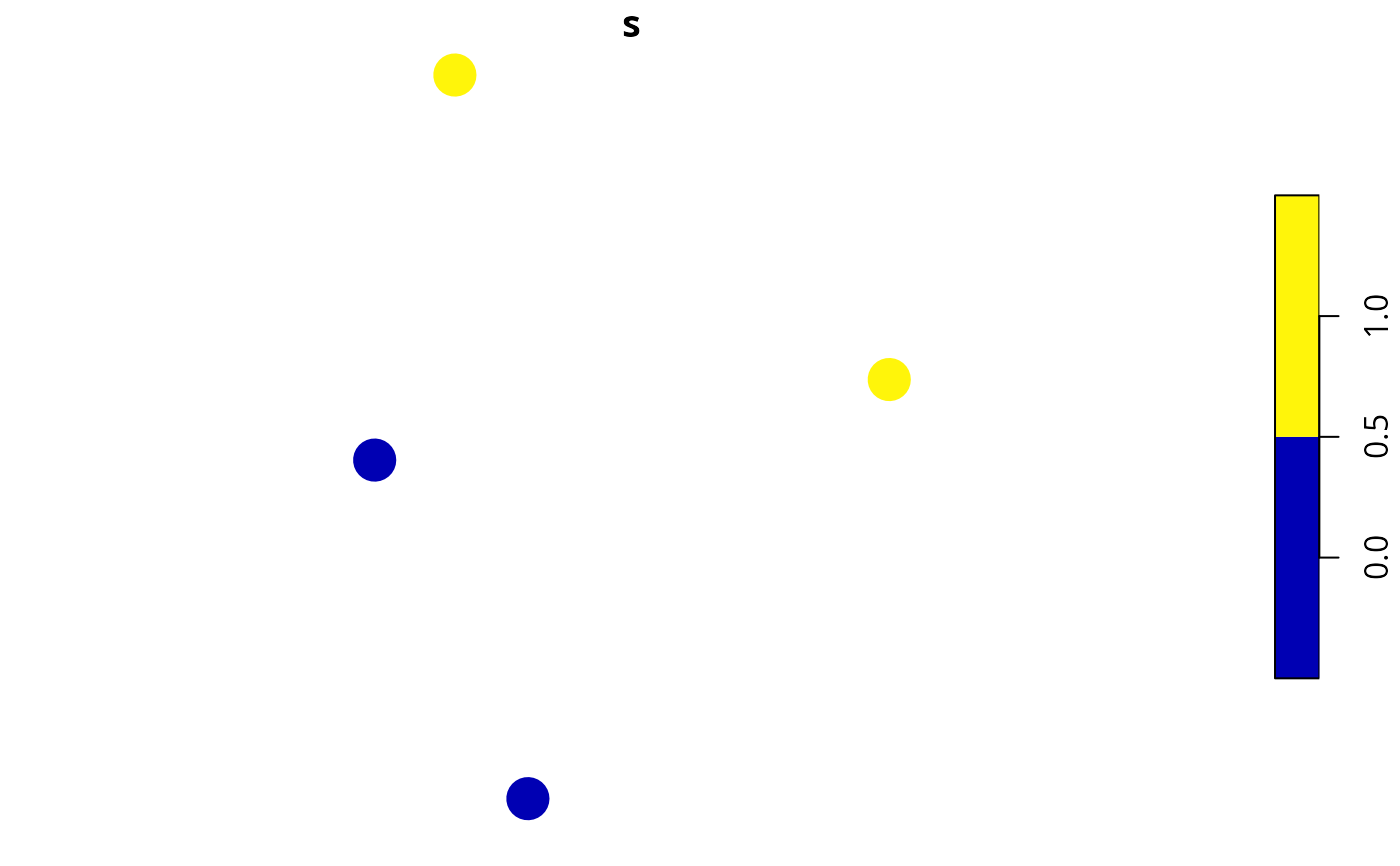Generate a survey scheme by selecting the set of sites with the greatest overall weight value, a maximum budget for the survey scheme.

weighted_survey_scheme(
site_data,
cost_column,
survey_budget,
weight_column,
locked_in_column = NULL,
locked_out_column = NULL,
solver = "auto",
verbose = FALSE
)

## Arguments

site_data

sf::sf() object containing the candidate survey sites.

cost_column

character name of the column in the argument to the argument to site_data that contains the cost for surveying each site. No missing (NA) values are permitted.

survey_budget

numeric vector of maximum budgets for the survey schemes. No missing (NA) values are permitted.

weight_column

character name of the column in the argument to site_data with the weights for each site.

locked_in_column

character (optional) name of the column in the argument to site_data that contains logical (TRUE/ FALSE) values indicating if certain sites should be locked into the survey scheme. No missing (NA) values are permitted. Defaults to NULL such that no sites are locked in.

locked_out_column

character (optional) name of the column in the argument to site_data that contains logical (TRUE/ FALSE) values indicating if certain sites should be locked out of the survey scheme. No missing (NA) values are permitted. Defaults to NULL such that no sites are locked out.

solver

character name of the optimization solver to use for generating survey schemes. Available options include: "Rsymphony", "gurobi" and "auto". The "auto" method will use the Gurobi optimization software if it is available; otherwise, it will use the SYMPHONY software via the Rsymphony package. Defaults to "auto".

verbose

logical indicating if information should be printed while generating survey scheme(s). Defaults to FALSE.

## Value

A matrix of logical (TRUE/ FALSE) values indicating if a site is selected in a scheme or not. Columns correspond to sites, and rows correspond to different schemes.

## Details

Let $$J$$ denote the set of sites (indexed by $$j$$), and let $$b$$ denote the maximum budget available for surveying the sites. Next, let $$c_j$$ represent the cost of surveying each site $$j \in J$$, and $$w_j$$ denote the relative value (weight) for surveying each site $$j \in J$$. The set of sites with the greatest overall weight values, subject to a given budget can the be identified by solving the following integer programming problem. Here, $$x_j$$ is the binary decision variable indicating each if site is selected in the survey scheme or not.

$$\mathit{Maximize} \space \sum_{j \in J} x_j w_i \\ \mathit{subject \space to} \\ \sum_{j \in J} x_j c_j \leq b$$

## Solver

This function can use the Rsymphony package and the Gurobi optimization software to generate survey schemes. Although the Rsymphony package is easier to install because it is freely available on the The Comprehensive R Archive Network (CRAN), it is strongly recommended to install the Gurobi optimization software and the gurobi R package because it can generate survey schemes much faster. Note that special academic licenses are available at no cost. Installation instructions are available online for Linux, Windows, and Mac OS operating systems.

## Examples

# set seed for reproducibility
set.seed(123)

# simulate data
x <- sf::st_as_sf(
tibble::tibble(x = rnorm(4), y = rnorm(4),
w = c(0.01, 10, 8, 1),
cost = c(1, 1, 1, 1)),
coords = c("x", "y"))

# plot site' locations and color by weight values
plot(x[, "w"], pch = 16, cex = 3)# generate scheme without any sites locked in
s <- weighted_survey_scheme(x, cost_column = "cost", survey_budget = 2,
weight_column = "w")

# print solution
print(s)
#>       [,1] [,2] [,3]  [,4]
#> [1,] FALSE TRUE TRUE FALSE

# plot solution
x\$s <- c(s)
plot(x[, "s"], pch = 16, cex = 3)